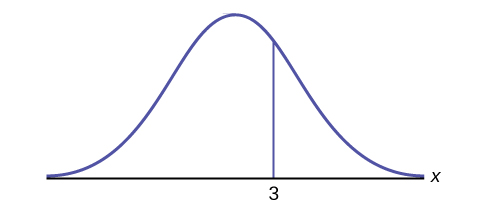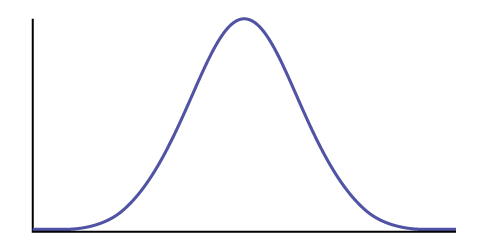# 6.3E: Using the Normal Distribution (Exercises)

•• OpenStax
• OpenStax
$$\newcommand{\vecs}{\overset { \rightharpoonup} {\mathbf{#1}} }$$ $$\newcommand{\vecd}{\overset{-\!-\!\rightharpoonup}{\vphantom{a}\smash {#1}}}$$$$\newcommand{\id}{\mathrm{id}}$$ $$\newcommand{\Span}{\mathrm{span}}$$ $$\newcommand{\kernel}{\mathrm{null}\,}$$ $$\newcommand{\range}{\mathrm{range}\,}$$ $$\newcommand{\RealPart}{\mathrm{Re}}$$ $$\newcommand{\ImaginaryPart}{\mathrm{Im}}$$ $$\newcommand{\Argument}{\mathrm{Arg}}$$ $$\newcommand{\norm}{\| #1 \|}$$ $$\newcommand{\inner}{\langle #1, #2 \rangle}$$ $$\newcommand{\Span}{\mathrm{span}}$$ $$\newcommand{\id}{\mathrm{id}}$$ $$\newcommand{\Span}{\mathrm{span}}$$ $$\newcommand{\kernel}{\mathrm{null}\,}$$ $$\newcommand{\range}{\mathrm{range}\,}$$ $$\newcommand{\RealPart}{\mathrm{Re}}$$ $$\newcommand{\ImaginaryPart}{\mathrm{Im}}$$ $$\newcommand{\Argument}{\mathrm{Arg}}$$ $$\newcommand{\norm}{\| #1 \|}$$ $$\newcommand{\inner}{\langle #1, #2 \rangle}$$ $$\newcommand{\Span}{\mathrm{span}}$$$$\newcommand{\AA}{\unicode[.8,0]{x212B}}$$

Exercise $$\PageIndex{7}$$

How would you represent the area to the left of one in a probability statement?Figure $$\PageIndex{8}$$.

$$P(x < 1)$$

Exercise $$\PageIndex{8}$$

Is $$P(x < 1)$$ equal to $$P(x \leq 1)$$? Why?

Yes, because they are the same in a continuous distribution: $$P(x = 1) = 0$$

Exercise $$\PageIndex{9}$$

How would you represent the area to the left of three in a probability statement?Figure $$\PageIndex{10}$$.

Exercise $$\PageIndex{10}$$

What is the area to the right of three?Figure $$\PageIndex{11}$$.

$$1 – P(x < 3)$$ or $$P(x > 3)$$

Exercise $$\PageIndex{11}$$

If the area to the left of $$x$$ in a normal distribution is 0.123, what is the area to the right of $$x$$?

Exercise $$\PageIndex{12}$$

If the area to the right of $$x$$ in a normal distribution is 0.543, what is the area to the left of $$x$$?

$$1 - 0.543 = 0.457$$

Use the following information to answer the next four exercises:

$$X \sim N(54, 8)$$

Exercise $$\PageIndex{13}$$

Find the probability that $$x > 56$$.

Exercise $$\PageIndex{14}$$

Find the probability that $$x < 30$$.

0.0013

Exercise $$\PageIndex{15}$$

Find the 80th percentile.

Exercise $$\PageIndex{16}$$

Find the 60th percentile.

56.03

Exercise $$\PageIndex{17}$$

$$X \sim N(6, 2)$$

Find the probability that $$x$$ is between three and nine.

Exercise $$\PageIndex{18}$$

$$X \sim N(–3, 4)$$

Find the probability that $$x$$ is between one and four.

0.1186

Exercise $$\PageIndex{19}$$

$$X \sim N(4, 5)$$

Find the maximum of $$x$$ in the bottom quartile.

Exercise $$\PageIndex{20}$$

Use the following information to answer the next three exercise: The life of Sunshine CD players is normally distributed with a mean of 4.1 years and a standard deviation of 1.3 years. A CD player is guaranteed for three years. We are interested in the length of time a CD player lasts. Find the probability that a CD player will break down during the guarantee period.

1. Sketch the situation. Label and scale the axes. Shade the region corresponding to the probability.Figure $$\PageIndex{12}$$.

$$P(0 < x <$$ ____________$$) =$$ ___________ (Use zero for the minimum value of $$x$$.)

1. Check student’s solution.
2. 3, 0.1979

Exercise $$\PageIndex{21}$$

Find the probability that a CD player will last between 2.8 and six years.

1. Sketch the situation. Label and scale the axes. Shade the region corresponding to the probability.Figure $$\PageIndex{13}$$.

$$P($$__________ $$< x <$$ __________$$)$$ = __________

Exercise $$\PageIndex{22}$$

Find the 70th percentile of the distribution for the time a CD player lasts.

1. Sketch the situation. Label and scale the axes. Shade the region corresponding to the lower 70%.Figure $$\PageIndex{14}$$.

$$P(x < k) =$$ __________ Therefore, $$k =$$ _________